# What Is Strain?

This section introduces Poisson’s ratio and stress so as to explain “strain” in terms of strain measurement.

## What is strain?

The topic of “strain” (ε) always comes up when considering the strength and structure of a product.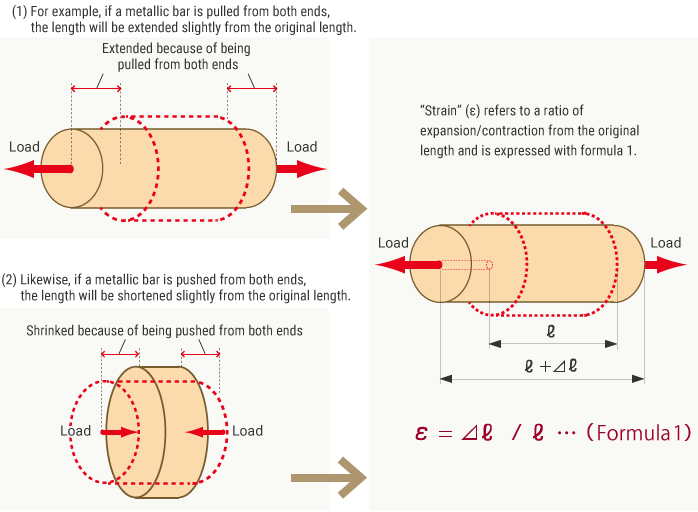Unit of strain

There is no unit for strain because it represents an expansion (contraction) as a ratio. In other words, it is treated as a “unitless” number. However, to indicate that “a given number represents a strain,” “ST” (an abbreviation of strain) or “ε” (because strain is generally represented by the Greek letter ε) is appended after the number. (This is the same way of thinking that is used with “%” and “ppm.”)Because strain represents a small value, micro strain is expressed with the prefix “μ” (micro, 1 × 10−6) as in “μST” or “με.”

## Poisson’s ratio and stress

If you pull a bar, its diameter will decrease as the bar expands. Strain in the direction of expansion (contraction) is called “longitudinal strain” while the change in the diameter direction (the direction perpendicular to the external force) is called “lateral strain” (εh).

1) Longitudinal strain is a ratio in the direction of expansion (contraction) of an object.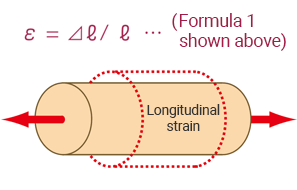2) Lateral strain is a ratio of the change in the diameter direction.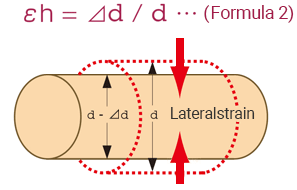The ratio between longitudinal and lateral strains is called the “Poisson’s ratio,” and general metallic materials have a ratio around 0.3.

ν = ｜εh/ε｜ — Formula 3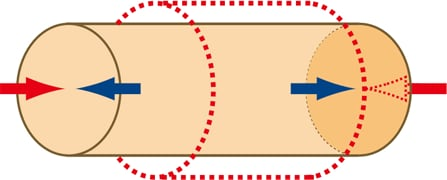Then, what force is working in the bar being pulled? In the bar being pulled, a force to return to the original shape is working (the magnitude is the same as that of the pulling force). Due to this working force, the bar will return to the original shape if you stop pulling it.

The value obtained by dividing this repelling force by the cross-sectional area (the value converted to per unit area) is called “stress” (σ). With the external pulling force as P (N) and the cross-sectional area as a (m2), the stress is obtained as follows.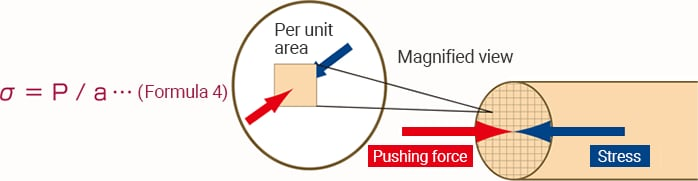Does strain have a direction (sign)?

Strain also has a direction and the plus or minus sign is attached to express the direction of expansion or contraction.
Pulling (expansion): plus, contraction: minus

## Relationship between stress and strain

The relationship between stress and strain has been obtained experimentally.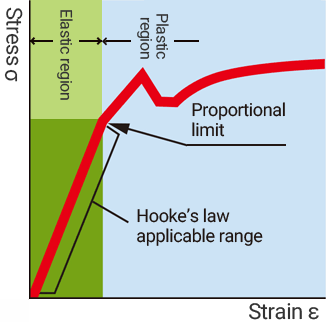Let’s take a metallic bar as an example. If it is bent only slightly, the bar will return to the original straight state. However, if the bar is bent forcefully, it will not return to the original state but will remain bent. The amount of bending (strain amount) that allows for a return to the original state is called the “elastic region” and the region above it is called the “plastic region.” In the elastic region, there is a linear relationship between stress and strain, which is known as “Young’s modulus” or the “modulus of longitudinal elasticity” and is generally expressed as “E.”
Once Young’s modulus (the modulus of longitudinal elasticity) is known, you can calculate the stress from the strain amount.

σ = (material-based constant E) × ε — Formula 5

Let’s obtain the stress (the applied force) from the strain amount.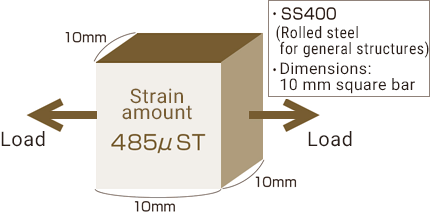Let’s obtain the stress by assuming that a strain of 485 μST has been measured after pulling the steel bar in the figure.

Conditions: Young’s modulus (the modulus of longitudinal elasticity) of SS400 E = 206 GPa, 1 Pa = 1 N/m2
From formula 5:
σ = E × ε = 206 GPa × 485 μST
= (206 × 109) × (485 × 10−6) = 99.9 MPa
From formula 4:
P = σ × a = 99.9 MPa × (0.01 m × 0.01 m)
= (99.9 × 106) × (1 × 10−4) = 9.99 kN
= Approximately 10 tons
Hence, it is assumed that the bar has been pulled with a load of approximately 10 tons.

### Strain gauges

Strain gauges use the principle that the resistance of a metal changes with its expansion/contraction.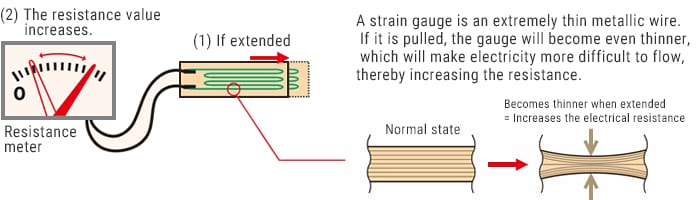With the original resistance as R (σ), the change in the resistance as ΔR (σ), and the strain amount as ε, this principle is expressed as follows.

ΔR / R = constant of proportionality K × ε — Formula 6

The constant of proportionality K is called the “gauge factor,” which has been determined on the basis of the metal (alloy) used for the strain gauge. With no load, the resistance of a strain gauge is generally 120 σ. During general strain measurement, the change in the resistance is several σ at most, so you need to take specific measures to achieve accurate strain measurement.

Unit of strain

Let’s obtain the stress (the applied force) from the strain amount. Here, it is assumed that the strain amount is 485 μST, the resistance of the strain gauge is 120 σ, and the gauge factor is 2.00. From formula 6:
ΔR = 2.00 × 485 μST × 120 σ = 0.1164 σ
Surprisingly, the resistance has changed only by 0.1164 σ. The change is as subtle as this.

INDEX